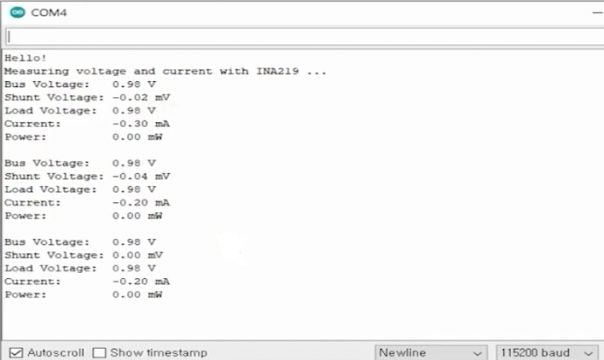Arduino Projects

# INA219 Current Sensor with Arduino circuit and code explained

## INA219 Current Sensor with Arduino:

In this article we will study about the INA219 Current Sensor, with this module we can measure current, voltage, and power of a circuit. The INA219 Current Sensor is an I2C supported interface based zero drift and bi-directional current/power monitoring module. The INA219 Current Sensor can be easily used with Arduino to measure current, power, and it can also sense shunt voltage. This Sensor module is provided with 0.1 ohms, a 1% shunt resistor to fulfill the requirement of current measurements. The INA219 Current Sensor can measure DC voltage up to +26V. There are so many other things which we will discuss in a minute. Without any further delay, let’s get started!!!

## Related Articles:

MAX471 Current Sensor

Overload Monitoring with ACS712 Current Sensor

INA219 Current Sensor

Arduino Uno

Arduino Nano

Other Tools and Components:

Top Arduino Sensors:

Super Starter kit for Beginners

Digital Oscilloscopes

Variable Supply

Digital Multimeter

Soldering iron kits

PCB small portable drill machines

## INA219 Current Sensor Pinout: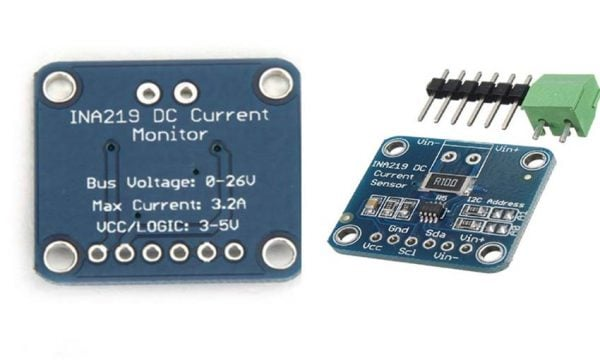The INA219 Current Sensor consists of six pins which are:

• VCC
• GND
• SCL
• SDA
• Vin –
• Vin +

In these pins the vcc and gnd pins are used for the power supply. SCL and SDA for the i2c communication and Vin+ and Vin- pins for measuring the voltage. On the board you can see A0 and A1 these are used for the I2C Address selection. The R100 is the Current Sense Resistor and the small chip in the middle is the INA219 chip. The INA219 Current Sensor is most commonly used:

• Power Profier.
• Digital Multimeter.

## Specifications of the INA219 Current Sensor:

### Supply voltage:

The supply voltage range is 3 to 5.5 volts so we can connect the supply to this module directly from our 5 volt or 3.3 volt systems. Due to this wide voltage input range the INA219 Current Sensor module can be powered up using the Arduino’s 5 volts. This current sensor module can also be easily interfaced with 3.3v compatible controller boards like ESP32 and Nodemcu ESP8266, etc.

### Shunt voltage and Bus voltage:

V_shunt, this is the voltage across the shunt resistor.V_shunt and  V_bus are the two measurements acquired directly by this module . The measurement range of these voltages is configurable by programming the registers through the i2c channel. The current measurement range can be determined by these settings and the value of the resistor we use for the shunt resistor. For example if we use a shunt resistor value of 0.1 ohm as in our module, the current measurement range are asshown here:

-0.4 A to 0.4 A

-0.8 A to 0.8 A

-1.6 A to 1.6 A

-3.2 A to 3.2 A

The resolution of the measurement is also programmable and has a range of 9 to 12 bits.

## INA219 Module Schematic:

This is the schematic of the module as you can see: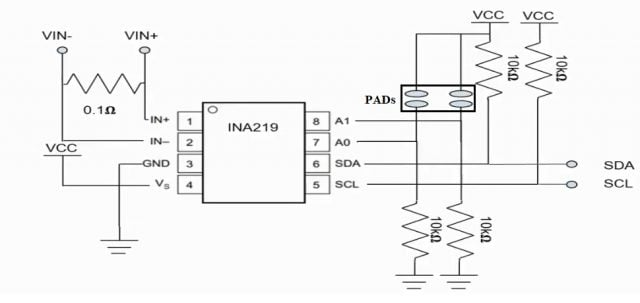It is composed of the INA219 IC with some resistors and one capacitor for noise decoupling but i omitted this for simplicity. Let’s discuss each component one by one, first there are two pull-down resistors connected to the address pins A0 and A1 which are 10 kilo ohm resistors and by default this device will have an address of 40 and hexadecimal value you can change this address by connecting these two pads as shown in the above figure. You can tie these pins to SDA or SCL extending your address options to anumber of 16.This is the list of the i2c addresses we can use for each connections made.

 A1 A0 Slave Address GND GND 1000000 GND 1000001 GND SDA 1000010 GND SCL 1000011 GND 1000100 1000101 SDA 1000110 SCL 1000111 SDA GND 1001000 SDA 1001001 SDA SDA 1001010 SDA SCL 1001011 SCL GND 1001100 SCL 1001101 SCL SDA 1001110 SCL SCL 1001111

The two pull-up resistors on the i2c line which are connected at SDA and SCL are necessary for proper communication with the host. Since i2c devices are only capable of driving the line to a low value and finally the resistor which is connected with the Vin– and Vin+ is called shunt resistor. It is used to measure the voltage drop or the shunt voltage and with the knowledge of the shunt resistor value. We can calculate the amount of current flowing to the load.

Let me show you an example in case we have a simple load connected to vcc and we want to measure the voltage and the amount of current flowing through this load, then we can connect the vin+ and Vin- pins of our module.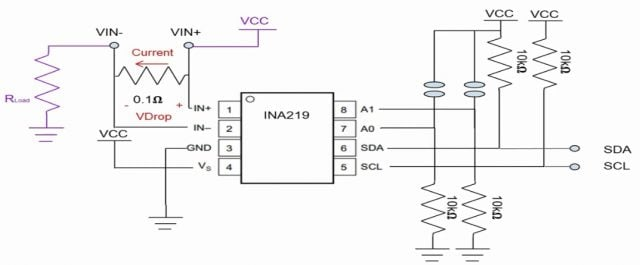As we see here and then the current flows through the shunt resistor which results in the voltage drop across the shunt resistor. So this voltage drop is:

V_shunt = Vdrop

V_bus = Vin –

## INA219 Register Description: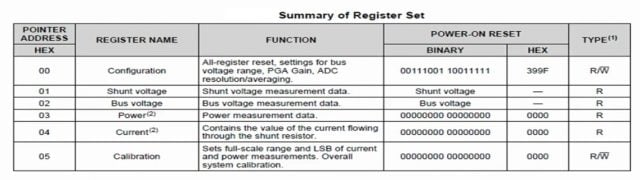Let’s take a look at the internal register of INA219, as you can see there are six registers, two configurable registers, and fou rread-only registers; with the configuration register we can set the mode of operation voltage measurement range ADC resolution and so on. The calibration register is used current and power calculations which we can read from the current and power registers. Current and power flowing to the load can be calculated using these formulas directly from the values read from the shunt and bus voltages registers.

Current (A) = (Shunt VoltageeReg * 10 μV ) / shunt Resistor

Power (W) = current * Bus voltage Reg * 4 mV

However you can also obtain current and power values directly from the registers after setting the calibration register using this formula:

Current value = 0.04096 (Current LSB * Rshunt)

current lsb is the desired resolution of the current register value and should be selected a value between maximum expected current divided by 2 to the 15th to maximum expected current divided by 2 to the 12th and of course the maximum expected current should be equal to or smaller than the current measurement range which was mentioned above. After setting the calibration register you can read the register value from the current and power register and obtain an actual value in amperes and watts using these formulas:

current (A) = Current Reg * Current LSB

Power (W) = Power Reg  * 20 * current LSB

For example with the maximum expected current of 2 amperes and desired resolution of the current as 100microamperes.

## Interfacing the INA219 with the Arduino:

Now for interfacing the INA219 Current Sensor with the arduino UNO we require the following components.

### Components Required:

• Arduino UNO or Arduino Nano
• INA219 current resistor
• Led
• Push button
• 180 ohm resistor

### INA219 connection with Arduino: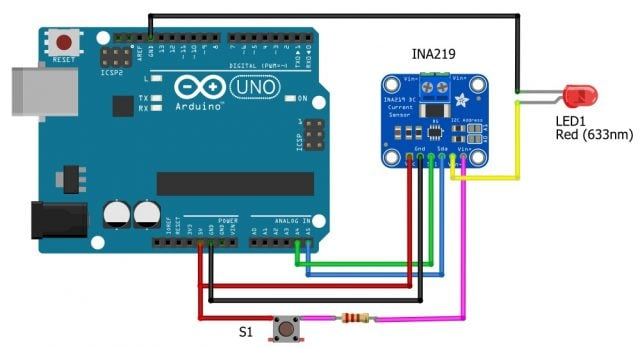Now to connect the arduino UNO with the INA219 current sensor we will perform the following steps:

• Connect the SDA pin of the INA219 current sensor with the A4 of the Arduino Uno
• Connect the SCL pin of the INA219 with the A5 of the Arduino Uno
• Connect the VCC pin of the INA 219 with 5V of the Arduino UNO
• Connect the ground pin of the INA219 current sensor with the ground of the Arduino UNO
• Connect one terminal of the push button with the 5V of the Arduino and connect the other terminal of the push button with the resistor
• Now connect the other terminal of the resistor with the Vin+
• Connect the cathode of the led with the ground and connect the anode of the led with the Vin-

Now let’s take a look at the schematic I used for testing the INA219 module.I used an Arduino UNO for thecontroller and for the load i used one led in series with a resistor for current limiting and added a push switch for turning on and off the led to show you the change of the current and voltage value. This is the connection example based on previous schematic.

### Demonstration of the INA219 with the Arduino:

So let’s start the demo; first start the Arduino IDE and make sure you have all your settings correctly applied in my case i am using the arduino uno as i mentioned before. When you have all your settings done you need to install the library. So click the manage libraries under the tools menu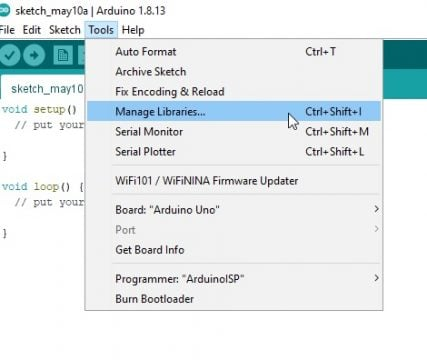Then type INA219 then press enter. You will see there are several libraries you can install but the one we are using is the top most one from adafruit, click the install button to install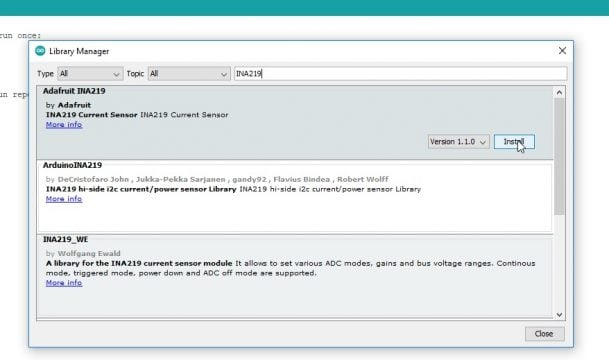And you may see a popup telling you there are dependencies to this library.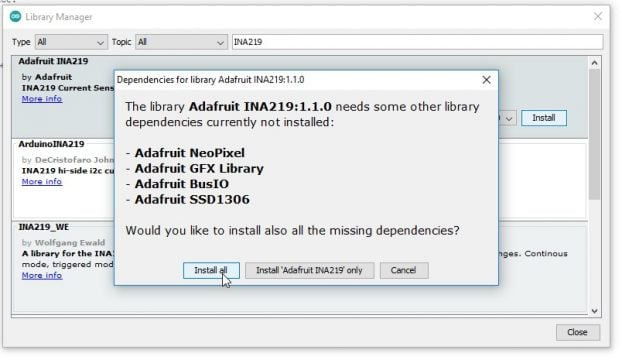So install them as well and then close.

After that you can open an example file, under file examples adafruit INA219 getcurrent. For testing purpose,  you don’t need to modify anything in this file.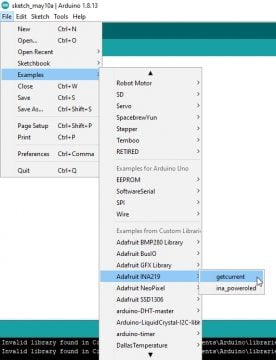## INA219 current sensor Arduino Code:

```#include <Wire.h>

void setup(void)
{
Serial.begin(115200);
while (!Serial) {
// will pause Zero, Leonardo, etc until serial console opens
delay(1);
}

uint32_t currentFrequency;

Serial.println("Hello!");

// Initialize the INA219.
// By default the initialization will use the largest range (32V, 2A).  However
// you can call a setCalibration function to change this range (see comments).
if (! ina219.begin()) {
Serial.println("Failed to find INA219 chip");
while (1) { delay(10); }
}
// To use a slightly lower 32V, 1A range (higher precision on amps):
//ina219.setCalibration_32V_1A();
// Or to use a lower 16V, 400mA range (higher precision on volts and amps):
//ina219.setCalibration_16V_400mA();

Serial.println("Measuring voltage and current with INA219 ...");
}

void loop(void)
{
float shuntvoltage = 0;
float busvoltage = 0;
float current_mA = 0;
float power_mW = 0;

shuntvoltage = ina219.getShuntVoltage_mV();
busvoltage = ina219.getBusVoltage_V();
current_mA = ina219.getCurrent_mA();
power_mW = ina219.getPower_mW();
loadvoltage = busvoltage + (shuntvoltage / 1000);

Serial.println("Bus Voltage:   ");
Serial.println(busvoltage);
Serial.println(" V");
Serial.println("Shunt Voltage: ");
Serial.println(shuntvoltage);
Serial.println(" mV");
Serial.println(" V");
Serial.print("Current:       ");
Serial.print(current_mA); Serial.println(" mA");
Serial.print("Power:         "); Serial.print(power_mW); Serial.println(" mW");
Serial.println("");

delay(2000);
}
```

So, I will just click upload, by default in this demo file, the address is 40 which is the i2c address. When you have all your address pins tied to the ground configuration register is not modified. So, it will have the default value of 399 F and hexadecimal value and you can take a look at the datasheet for the details calibration register is initialized to 4096 in decimal value as we have seen in the previous example in the register descriptionwith maximum expected current of 2 amperes and current lsb to 100 microamperes if you want to change the settings you can uncomment these lines for several pre-defined configurations.

// To use a slightly lower 32V, 1A range (higher precision on amps):

//ina219.setCalibration_32V_1A();

// Or to use a lower 16V, 400mA range (higher precision on volts and amps):

//ina219.setCalibration_16V_400mA()

After uploading the code, click serial monitor under tools and you can see the measurement result printed every two seconds. When i press the push switch led turns on and you can see the current andvoltage of the load, if the switch is released the led turns off and the current value returns tozero, if you are not able to see the printed value make sure your baud rate is set to115200.